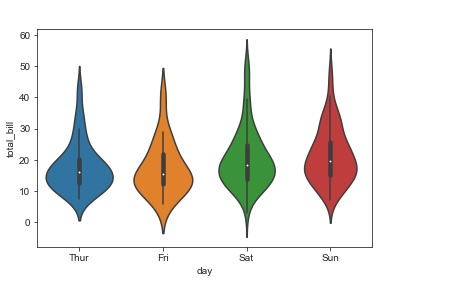# Demonstrate the working of violin plots in Python?

If we wish to compare the data present within categories, box plots come into play. It is a way in which the data distribution in the dataset can be understood with the help of quartiles. It consists of vertical lines that extend from the boxes. These extensions are known as whiskers. These whiskers tells about how the data varies outside the upper and lower quartiles. This is why box plots are also known as whisker plots. Outliers in the data are plotted as individual points.

Violin plot is a combination of box plot with kernel density estimates (KDE). It is easier to analyse and understand how the data has been distributed. The wide portion of the violin indicates the higher density of data. The narrow portion of the violin indicates the lower density of data.

The inter-quartile range within a boxplot and the higher density portion of data fall within the same region in every category.

Syntax of violinplot function

seaborn.violinplot(x, y,data,…)

Let us understand how a violin plot can be used to plot data −

## Example

import pandas as pd
import seaborn as sb
from matplotlib import pyplot as plt
sb.violinplot(x = "day", y = "total_bill", data=my_df)
plt.show()

## Output## Explanation

• The required packages are imported.
• The input data is ‘iris_data’ which is loaded from the scikit learn library.
• This data is stored in a dataframe.